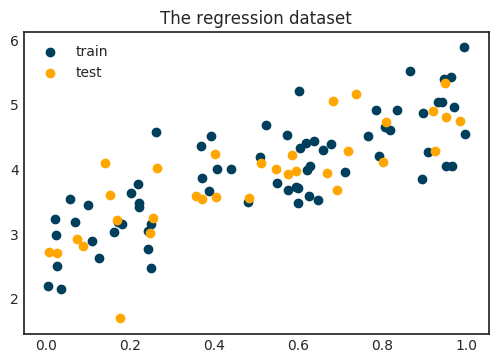# Where does the Mean Squared Error come from?The simplest and one of the most commonly used loss functions in machine learning is the mean squared error, defined by

$\text{MSE}(\mathcal{D}, f) = \frac{1}{n} \sum_{i=1}^{n} | y_i - f(x_i) |^2$

which is just the mean of the squared distances between ground truth and prediction.

The mean squared error conveys a completely natural idea. Intuitively, the distance between ground truth and prediction should indicate how well our model fits the data. The closer they are, the better the fit.

Beyond this intuition, mean squared error has its roots in probability theory. For one, as the observations yᵢ can be thought of as samples from a probability distribution, MSE is the empirical expected value of

$| y_i - f(x_i) |^2.$

Minimizing the MSE is the same as minimizing the expected value of the error between prediction and ground truth. However, this interpretation does not offer an insight into why such a model would explain the dataset. Consider the following simple example below.Notice that around each $\textstyle x$, the observations $\textstyle y$ fluctuate and exhibit high variance. It seems like that a deterministic model $y = f(x)$ is not enough to "explain" the data. Instead of fitting a function, let's look for a model that also accounts for the variance in the dataset. Finding a parametrized function like $f(x) = ax + b$ is not good enough. We need a model that can explain the variance of the data, not just its mean, unlike a linear regression would. So, let's model it with probability distributions instead of just a function!

## Mean squared error as maximum likelihood

To explore this idea, let's go back to a simple special case and suppose that both our input and output are a single number. Say we are trying to predict the price of an apartment from its size in square foot.

Suppose that the data $\textstyle x$ and the observation $\textstyle y$ comes from the distributions $\textstyle X$ and $\textstyle Y$. What we are looking for is the conditional distribution of $\textstyle Y$ given $\textstyle X$. As it is a probability distribution, it provides a more refined understanding of the underlying process.

How can we approximate it? For one we can model it with Gaussian distributions, so that

$P_Y(y | X = x, f) = \mathcal{N}(y | f(x), \sigma^2),$

where $f(x)$ describes the expected value function. To fit such a model, we can turn to maximum likelihood estimation. Here, the maximum likelihood function is

$\text{L}(f, \mathbf{x}, \mathbf{y}) = \prod_{i=1}^{n} P_{Y_i}(y_i | f, x_i).$

After a bit of calculation, we obtain

\begin{align*} \text{L}(f, \mathbf{x}, \mathbf{y}) &= \prod_{i=1}^{n} P_{Y_i}(y_i | f, x_i) \\ &= \prod_{i=1}^{n} \mathcal{N}(y_i | f(x_i), \sigma^2) \\ &= \prod_{i=1}^{n} \frac{1}{\sigma \sqrt{2 \pi}} e^{- \frac{(y_i - f(x_i))^2}{2 \sigma^2}} \end{align*}

This is what we want to maximize. Now we do a trick that should be familiar by now: maximizing a function is the same as maximizing its logarithm. (Since the logarithm is monotone increasing.)

The purpose of this is to turn the product into a sum:

\begin{align*} \text{L}(f, \mathbf{x}, \mathbf{y}) &= \sum_{i=1}^{n} \bigg[ \log \frac{1}{\sigma \sqrt{2 \pi}} - \frac{1}{2\sigma^2} \big( y_i - f(x_i) \big)^2 \bigg]^2 \\ &= n \log \frac{1}{\sigma \sqrt{2 \pi}} - \sum_{i=1}^{n} \frac{1}{2\sigma^2} \big( y_i - f(x_i) \big)^2 \end{align*}

Because we want to maximize the above formula in $\textstyle f$, we can omit the terms where it is not present. Moreover, maximizing a function is the same as minimizing its negative. In the end, we are left with

$\arg \max_{f} \text{L}(f, \mathbf{x}, \mathbf{y}) = \arg \max_{f} \sum_{i=1}^{n} \big( y_i - f(x_i) \big)^2.$

This is almost the mean squared error! One thing is missing, though: scaling with the number of samples.

## Taking the average of norms

The mean squared error is the mean of squared errors. Why not just take the sum? The reason is to keep the loss independent of the dataset size. Consider the two scenarios:

1. A model that fits poorly on a dataset with 10 samples.
2. A model that fits excellently on a dataset with 1000 samples.

Without scaling taking the mean, the sum of squared errors can be the same in both cases. Roughly speaking,

$\sum_{i=1}^{10} (\text{some large number})_i \approx \sum_{i=1}^{1000} (\text{some small number})_i,$

which is not something we would like. By scaling, we have

$\frac{1}{10} \sum_{i=1}^{10} (\text{some large number})_i > \frac{1}{1000} \sum_{i=1}^{1000} (\text{some small number})_i,$

indicating the fact that the second model fits better. There is an additional benefit: it keeps the gradient in check. For large datasets, the gradient can be huge, throwing the optimization off by forcing it to take giant steps.